# C++ Program to Calculate the LCM and HCF (GCD) of Two Numbers

In this article, you will learn and get code to find and print the LCM and HCF (GCD) of any given two numbers given by the user at run-time in C++. Here is the list of programs you will go through:

### How do LCM and HCF get calculated?

Before going through the programs listed above, if you're not aware of how the LCM or HCF of two numbers gets calculated, then you can refer to these articles:

to clear all your doubts about LCM and HCF. Now let's move on to the program.

## Determine the LCM of Two Numbers in C++

To find the LCF of two numbers in C++ programming, you have to ask the user to enter the two numbers. Then find and print its LCM on the output, as shown in the program given below.

Note: LCM is the Lowest Common Multiple (or Least Common Divisor). For example, if there are two numbers, say 10 and 12, then their least common divisor is 60. That is, both numbers 10 and 12 divide 60 without leaving any remainder (or leaving 0 as a remainder).

```#include<iostream>
using namespace std;
int main()
{
intnumOne, numTwo, mp;
cout<<"Enter Two Numbers: ";
cin>>numOne>>numTwo;
if(numOne>numTwo)
mp = numOne;
else
mp = numTwo;
while(1)
{
if((mp%numOne == 0) && (mp%numTwo == 0))
break;
else
mp++;
}
cout<<"\nLCM ("<<numOne<<", "<<numTwo<<") = "<<mp;
cout<<endl;
return 0;
}```

This program was built and runs under the Code::Blocks IDE. Here is its sample run: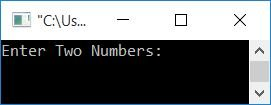Now supply any two numbers, say 2 and 3. Press the ENTER key to find and print its LCM, as shown in the output given below:Note: 6 is the lowest number that is completely divisible by both 2 and 3.

Here is another sample run with user input: 4, 5:The main logic behind the above program is:

• Sets the greater number to mp.
• Putting 1 as a condition of the while loop always evaluates to true. As a result, this loop will continue to execute until the break keyword is executed.
• The break keyword gets executed when both the condition of if (inside the while loop) evaluates to be true.
• Every time entering the while loop's body, a condition is applied using if, which checks whether the value in mp is divisible by both the number.
• If it is divisible, then use the break keyword and exit from the loop.
• Otherwise, increment its value and keep checking with next.

The dry run of the above program with user inputs 2 and 3 goes like this:

• When the user enters 2 and 3 as input. Then it gets stored in the numOne and numTwo variables. As a result, numOne=2 and numTwo=3.
• The condition of the if gets executed. That is, the condition numOne>numTwo or 2>3 evaluates to be false, therefore program flow does not go inside the if's body, but rather to its else's part, and the value of numTwo gets initialized to mp. So mp=3
• Now that condition 1 (of the while loop) evaluates to true, program flow goes inside the loop.
• Inside the loop, the condition of the if gets evaluated. That is the condition, mp%numOne==0, 3%2==0, or 1==0 evaluates to be false. Because the first condition evaluates to false, the second condition does need to be evaluated.
• So the value of mp gets incremented, and we check for the condition again with the new value of mp, which is 4 for the second time here.
• This process continues until both conditions are evaluated to be true, and program flow goes inside if's body. It then executes the break keyword to stop the execution of the while loop.
• When the value of mp becomes 6, then both conditions are satisfied, that is, 6 is divisible by both numbers, say 2 and 3.
• Therefore, after exiting the loop, the value of mp will be 6.
• Print the value of mp as the LCM result of the given two numbers.

## C++ Determine the HCF (GCD) of Two Numbers

Unlike LCM, which deals with multiples (the lowest common), HCM deals with factors (the highest common). HCF can be called the "highest common factor" (HCF) or "greatest common factor" (GCF).

For example, if there are two numbers, say 10 and 12, then their highest common factor is 2. That is, 2 is the highest number that divides both numbers. 1 also divides both numbers, but 2 is greater, so 2 is the HCF of 10 and  12.

The question is, "Write a program in C++ that finds the HCF of two numbers." Here is its answer:

```#include<iostream>
using namespace std;
int main()
{
intnumOne, numTwo, mp;
cout<<"Enter Two Numbers: ";
cin>>numOne>>numTwo;
if(numOne<numTwo)
mp = numOne;
else
mp = numTwo;
while(1)
{
if((numOne%mp == 0) && (numTwo%mp == 0))
break;
else
mp--;
}
cout<<"\nHCF ("<<numOne<<", "<<numTwo<<") = "<<mp;
cout<<endl;
return 0;
}```

Here is its sample run with user input (2 and 3):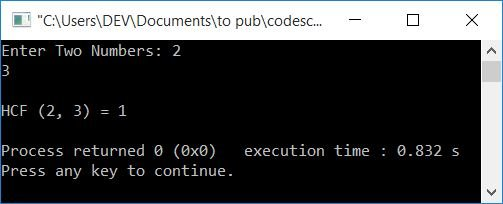Note: Because 1 is the highest positive integer that divides both the numbers 2 and 3, therefore, 1 is the HCF of 2 and 3.

Take note that the highest positive integer must be less than the smaller of the two numbers given.

Here is another sample run with user input, 18 and 27: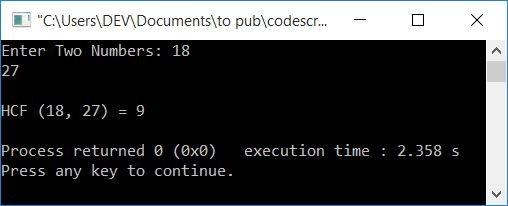Because 9 is the highest positive integer that divides both the numbers 18 and 27.

The logic used here is a little similar to the previous program on LCM of two numbers. The difference is in the condition and the update of the mp variable. That is, instead of incrementing it, decrement its value every time you enter the loop. And the condition gets changed with:

`if((numOne%mp == 0) && (numTwo%mp == 0))`

## C++ LCM and HCF in One Program

This C++ program receives two numbers from the user and finds both LCM and HCF.

```#include<iostream>
using namespace std;
int main()
{
intnumOne, numTwo, lcm, hcf, a, b, temp;
cout<<"Enter Two Numbers: ";
cin>>numOne>>numTwo;
a = numOne;
b = numTwo;
while(b!=0)
{
temp = b;
b = a%b;
a = temp;
}
hcf = a;
lcm = (numOne*numTwo)/hcf;
cout<<"\nLCM ("<<numOne<<", "<<numTwo<<") = "<<lcm;
cout<<"\nHCF ("<<numOne<<", "<<numTwo<<") = "<<hcf;
cout<<endl;
return 0;
}```

Here is its sample run with user input: 9, 8: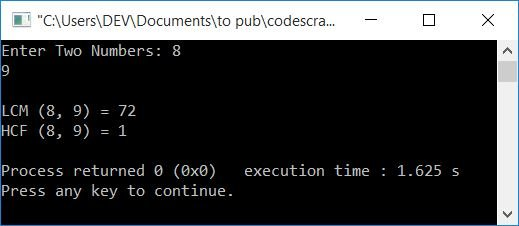## In C++, find the LCM and HCF of two numbers using a for loop

Now let's create the same program using the for loop.

```#include<iostream>
using namespace std;
int main()
{
intnumOne, numTwo, mp, temp;
cout<<"Enter Two Numbers: ";
cin>>numOne>>numTwo;
if(numOne>numTwo)
mp = numOne;
else
mp = numTwo;
temp = mp;
for(;;mp++)
{
if((mp%numOne == 0) && (mp%numTwo == 0))
break;
}
cout<<"\nLCM ("<<numOne<<", "<<numTwo<<") = "<<mp;
mp = temp;
for(;;mp--)
{
if((numOne%mp == 0) && (numTwo%mp == 0))
break;
}
cout<<"\nHCF ("<<numOne<<", "<<numTwo<<") = "<<mp;
cout<<endl;
return 0;
}```

Here is a sample run with user input: 10 and 12.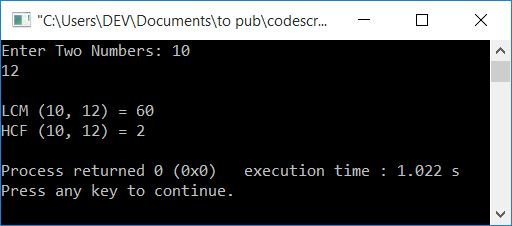NoteThe initialization part (first part) and condition-checking part (second part) of the for loop are hidden. Leaving the condition empty always evaluates to a true condition.

## In C++, find the LCM and HCF of two numbers using a user-defined function

This program uses two user-defined functions, namely, findLCM() and findHCF(), to find the LCM and HCF of two numbers entered by the user.

Both functions accept two arguments, a first and second number, and return the LCM or HCF of the two numbers.

```#include<iostream>
using namespace std;
int findLCM(int, int);
int findHCF(int, int);
int main()
{
intnumOne, numTwo, res;
cout<<"Enter Two Numbers: ";
cin>>numOne>>numTwo;
res = findLCM(numOne, numTwo);
cout<<"\nLCM ("<<numOne<<", "<<numTwo<<") = "<<res;
res = findHCF(numOne, numTwo);
cout<<"\nHCF ("<<numOne<<", "<<numTwo<<") = "<<res;
cout<<endl;
return 0;
}
int findLCM(int a, int b)
{
intlcm;
if(a>b)
lcm = a;
else
lcm = b;
while(1)
{
if((lcm%a == 0) && (lcm%b == 0))
break;
lcm++;
}
return lcm;
}
int findHCF(int a, int b)
{
inthcf;
if(a>b)
hcf = a;
else
hcf = b;
while(1)
{
if((a%hcf == 0) && (b%hcf == 0))
break;
hcf--;
}
return hcf;
}```

Here is its sample run with user input: 6 and 8.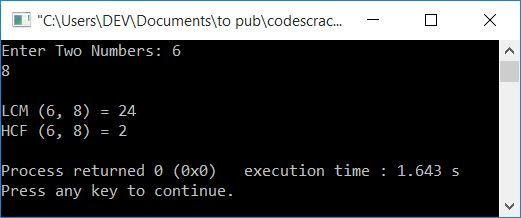#### The same program in different languages

C++ Quiz

« Previous Program Next Program »